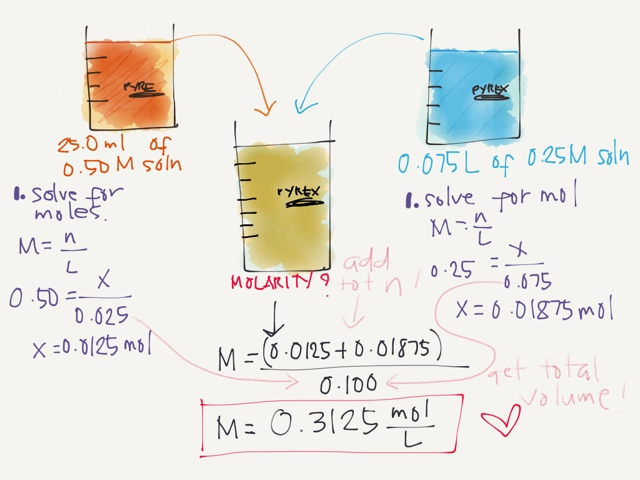# Question 636b6

Jul 17, 2014

Thanks for your question. Do you have three separate questions here?

We'll answer your questions from that standpoint.

Molarity = moles of solute$\div$ liters of solution

Your first step will be to determine the number of moles in each solution. Keep in mind that your volume needs to be in liters to work these problems, so the milliliters (mL) need to converted to liters (L)

Question 1: the HCl

M = moles of solute/Liters of solution

12 M = X$\div$.20000
X = 2.4 moles of HCl

Next, let's look at the total solution. When you add 200.0 mL of HCl to 200.0 mL of water, you have a total volume of 400.0 mL or .4000 L.

X = 2.4 moles HCl $\div$ .4000 L
X = 6.0 M HCl

Try this method on each problem. It will work!! And please let me know if you need more help.

Good luck!

Here's an example problem.

What is the molarity of a solution prepared by mixing 35.0 mL of 0.375mol/L NaCl solution with 47.5 mL of a 0.632 mol/L NaCl solution?

Step 1. Calculate the moles of NaCl in the first solution.

Moles of NaCl = 0.0350 L soln × $\left(0.375 \text{mol NaCl")/(1"L soln}\right)$ = 0.013 125 mol NaCl (3 significant figures + 2 guard digits)

Step 2. Calculate the moles of NaCl in the second solution.

Moles of NaCl = 0.0475 L soln × $\left(0.632 \text{mol NaCl")/(1"L soln}\right)$ = 0.030 020 mol NaCl

Step 3. Calculate the total moles of NaCl.

Moles of NaCl = (0.013 125 + 0.030 020) mol NaCl = 0.043 145 mol NaCl

Step 4. Calculate the total volume of the solution.

Volume = (35.0 + 47.5) mL = 82.5 mL = 0.0825 L

Step 5. Calculate the new molarity.

Molarity = "moles"/"litres" = (0.043 145"mol")/(0.0825"L") =0.523 mol/L (3 significant figures)